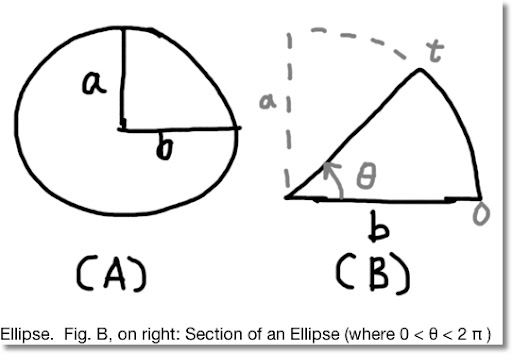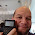## Wednesday, May 23, 2012

### Area of an EllipseBlog Entry #104. (I thought I use a different font this time. I like Arial, but change is good, at least every once in a while. )

Objective of this blog entry: calculate the area of an ellipse (Fig. A), and then a section of an ellipse (Fig. B).

Normally, the equation given for an ellipse is:

x^2/a^2 + y^2/b^2 = 1

I am going to use the parametric form

x = a cos t
y = b sin t
with 0 ≤ t ≤ 2 π

Let θ be the upper limit (angle) and the area is:

θ
∫ y dx =
0

θ
∫ y(t) x'(t) dt =
0

θ
∫ (b sin t) (a sin t) dt =
0

θ
∫ a b sin^2 t dt
0

Note: the antiderviatve of sin^2 t is 1/2 ( t - sin t cos t ).

Then:

θ
∫ a b sin^2 t dt =
0

a b [ 1/2 ( θ - sin θ cos θ ) - 1/2 ( 0 - sin 0 cos 0 ) ] =

1/2 a b ( θ - sin θ cos θ )

The area of an ellipse (up to angle θ , see Fig. B) is:
1/2 a b ( θ - sin θ cos θ )

To find the area of the entire ellipse, let θ = 2 π. Then:

A = 1/2 a b (2 π - sin (2 π) cos (2 π)) = π a b

That concludes is blog entry. Before I go, and before I forget: THANK YOU U.S. TROOPS FOR ALL YOU DO!!!!

Eddie

1.Thanks for providing such useful information about ellipse .The formulas and their derivation which are provided here are very useful.
Area of an Ellipse

2.You are welcome Manoj.

3.### Calculator Python: Lambda Functions

Calculator Python: Lambda Functions Introduction to Lambda Functions Lambda functions are a quick, one expression, one line, python function...